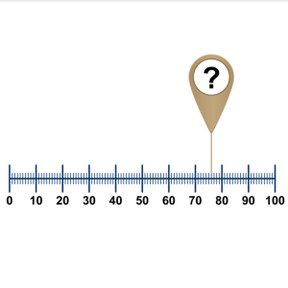Number line to 100 with random numbers

# Number line to 100 with random numbers

Number line to 100 with random numbers

No account needed.8,000 schools use Gynzy92,000 teachers use Gynzy1,600,000 students use Gynzy

## General

Students learn to place random numbers on a number line to 100. They count forwards and backward.

-1.NBT.B.2

## Introduction

The interactive whiteboard shows a number line from 0 to 100. Discuss with the students how we should place tens on the number line. Erase the boxes to show the tens. Have students write the remaining tens numbers. Next show students a counting sequence with a few missing numbers. Have them write the missing numbers on a sheet of paper, and then hold them up so you can check their answers. Erase the boxes on the interactive whiteboard to show the answers.

## Instruction

Explain what a number line is and say that every mark represents one number. On a number line from 0 to 100, you can quickly determine which number is at a question mark. First look to see which tens numbers the question mark is between. Then look to see which tens it is closest to. Count forwards or backward from that number to determine the number at the question mark. Practice together with students, asking which numbers belong at the question marks, and drag the numbers to the correct places.

Check that students understand placing random numbers to 100 on a number line by asking the following questions:
- What is a number line?
- How do you place numbers on a number line?
- Where do you start counting?
- When do you count forwards and when do you count backward?

## Quiz

Students practice placing numbers on the number line with counting forwards and backward. They practice this by using a number line with tens. Next, they practice on a number line without tens. There are multiple-choice questions and fill-in-the-blank questions.

## Closing

Discuss with students that it is important to be able to place numbers on a number line because you then know where a number belongs. You can quickly place a number by skip counting in tens to the closest ten and then counting forwards or backward from that number. To check that students have understood, show a number line on the interactive whiteboard. Have a few students come forward and place numbers on the number line.

## Teaching tips

Students who have difficulty placing random numbers on the number line to 100 can be supported by practicing counting forwards and backward to 100, as well as practicing skip counting in 10s to 100.

### The online teaching platform for interactive whiteboards and displays in schools

• Save time building lessons

• Manage the classroom more efficiently

• Increase student engagement